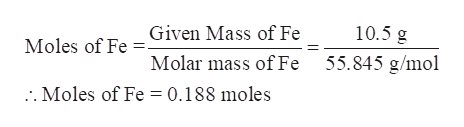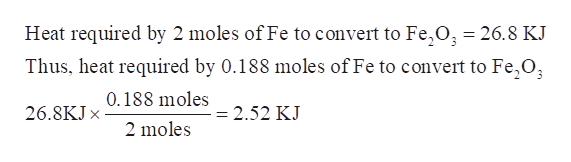# Iron can be converted to Fe2O3 according to the reaction below. How much heat (in kJ) is required to convert 10.5 g of iron to Fe2O3?2Fe(s) + 3CO(g) → Fe2O3(s) + 3CO2(g)  ΔH = 26.8 kJ/mol

Question

Iron can be converted to Fe2O3 according to the reaction below. How much heat (in kJ) is required to convert 10.5 g of iron to Fe2O3?

2Fe(s) + 3CO(g) → Fe2O3(s) + 3CO2(g)  ΔH = 26.8 kJ/mol

check_circle

Step 1

Iron can be converted to Fe2O3 according to the given reaction. Amount of heat (in kJ) required to convert 10.5 g of iron into Fe2O3 is to be calculated.

Step 2

Calculate the moles of Fe in 10.5 g of iron-

As per the balanced equation, 2 moles of Fe needs 26.8 KJ of heat to convert to Fe2O3.

Thus, first convert 10.5 g of Fe into moles of Fe.help_outlineImage TranscriptioncloseGiven Mass of Fe 10.5 g Moles of Fe 55.845 g/mol Molar mass of Fe . Moles of Fe = 0.188 moles fullscreen
Step 3

Calculate the amount of heat (in kJ) required to convert 0....help_outlineImage TranscriptioncloseHeat required by 2 moles of Fe to convert to Fe, O, = 26.8 KJ Thus, heat required by 0.188 moles of Fe to convert to Fe,0 0.188 moles 26.8KJX = 2.52 KJ 2 moles fullscreen

### Want to see the full answer?

See Solution

#### Want to see this answer and more?

Solutions are written by subject experts who are available 24/7. Questions are typically answered within 1 hour.*

See Solution
*Response times may vary by subject and question.
Tagged in

### Physical Chemistry